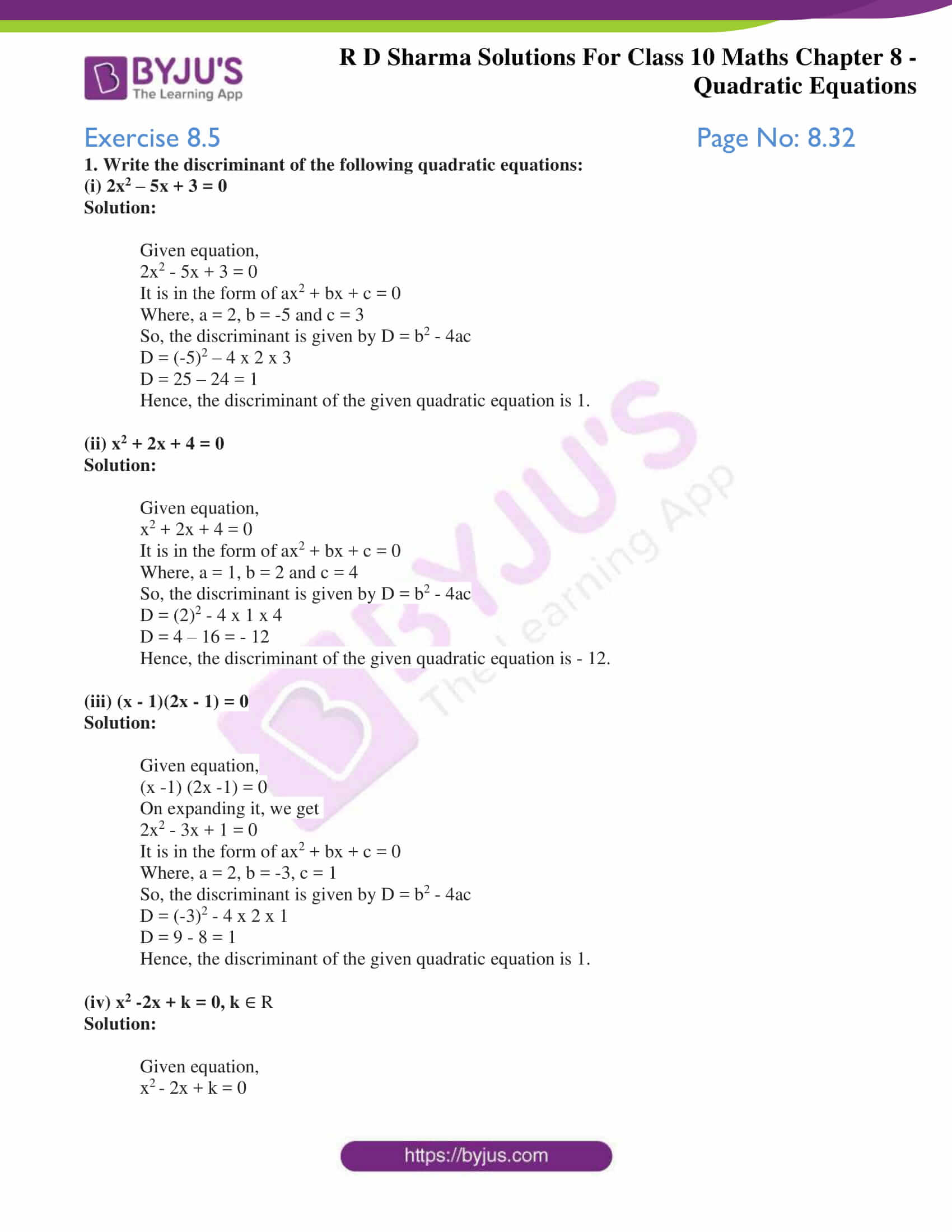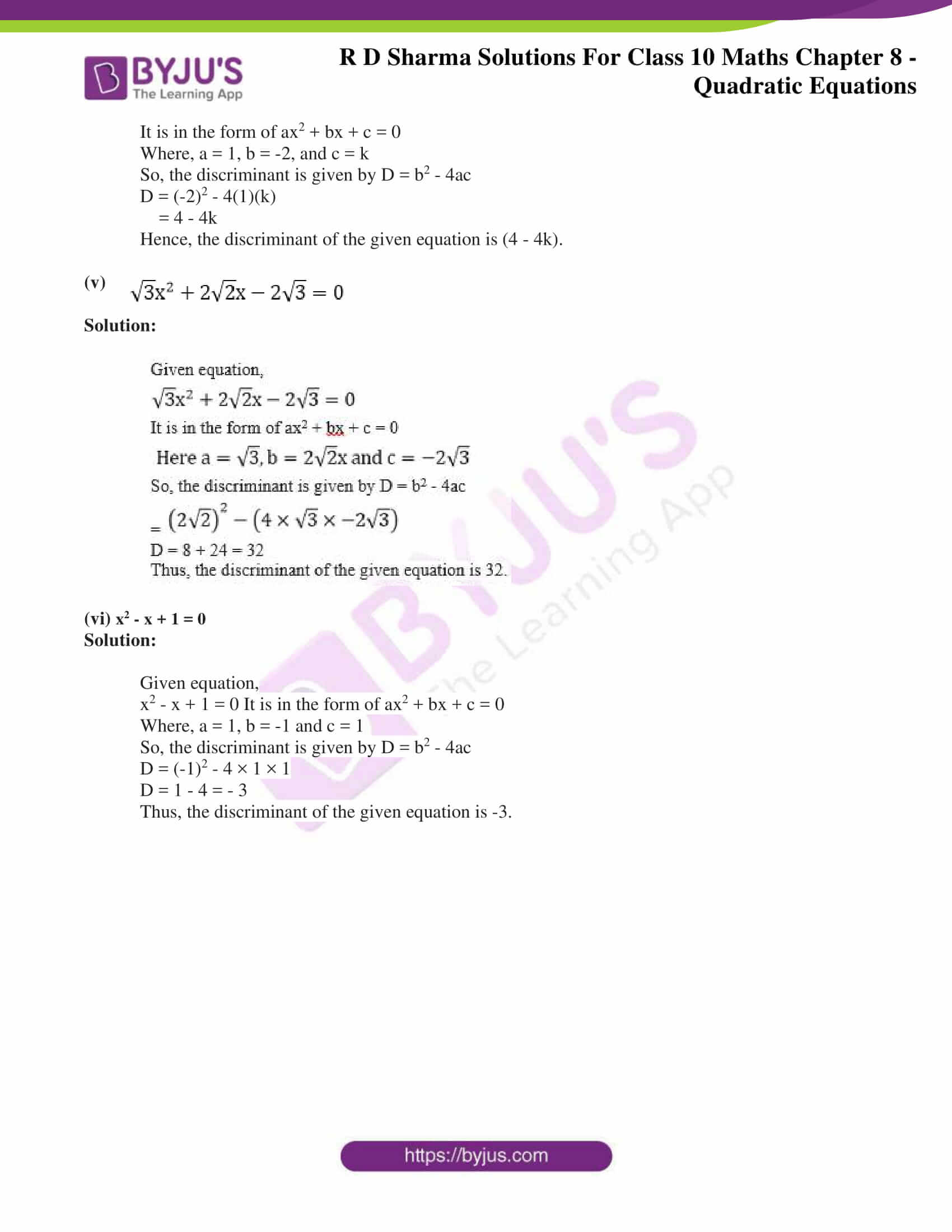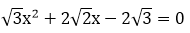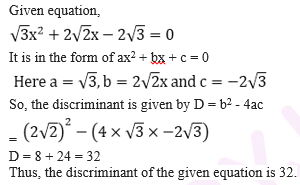# RD Sharma Solutions for Class 10 Maths Chapter 8 Quadratic Equations Exercise 8.5

Another method commonly called as the Quadratic formula is the main focus in this exercise. It’s also known as the Shreedharacharya’s rule. This method overcomes certain limitations of the factorization method. For detailed study regarding this concept, students can refer the RD Sharma Solutions Class 10 prepared by experts at BYJU’S. The RD Sharma Solutions for Class 10 Maths Chapter 8 Quadratic Equations Exercise 8.5 PDF is provided below.### Access RD Sharma Solutions for Class 10 Maths Chapter 8 Quadratic Equations Exercise 8.5

1. Write the discriminant of the following quadratic equations:

(i) 2x2 – 5x + 3 = 0

Solution:

Given equation,

2x2 – 5x + 3 = 0

It is in the form of ax2 + bx + c = 0

Where, a = 2, b = -5 and c = 3

So, the discriminant is given by D = b2 – 4ac

D = (-5)2 – 4 x 2 x 3

D = 25 – 24 = 1

Hence, the discriminant of the given quadratic equation is 1.

(ii) x2 + 2x + 4 = 0

Solution:

Given equation,

x2 + 2x + 4 = 0

It is in the form of ax2 + bx + c = 0

Where, a = 1, b = 2 and c = 4

So, the discriminant is given by D = b2 – 4ac

D = (2)2 – 4 x 1 x 4

D = 4 – 16 = – 12

Hence, the discriminant of the given quadratic equation is – 12.

(iii) (x – 1)(2x – 1) = 0

Solution:

Given equation,

(x -1) (2x -1) = 0

On expanding it, we get

2x2 – 3x + 1 = 0

It is in the form of ax2 + bx + c = 0

Where, a = 2, b = -3, c = 1

So, the discriminant is given by D = b2 – 4ac

D = (-3)2 – 4 x 2 x 1

D = 9 – 8 = 1

Hence, the discriminant of the given quadratic equation is 1.

(iv) x2 -2x + k = 0, k ∈ R

Solution:

Given equation,

x– 2x + k = 0

It is in the form of ax2 + bx + c = 0

Where, a = 1, b = -2, and c = k

So, the discriminant is given by D = b2 – 4ac

D = (-2)2 – 4(1)(k)

= 4 – 4k

Hence, the discriminant of the given equation is (4 – 4k).(v)

Solution:(vi) x2 – x + 1 = 0

Solution:

Given equation,

x2 – x + 1 = 0 It is in the form of ax2 + bx + c = 0

Where, a = 1, b = -1 and c = 1

So, the discriminant is given by D = b2 – 4ac

D = (-1)2 – 4 × 1 × 1

D = 1 – 4 = – 3

Thus, the discriminant of the given equation is -3.